# Fundamentals of ThermalFluid Sciences 4 th Edition in

• Slides: 31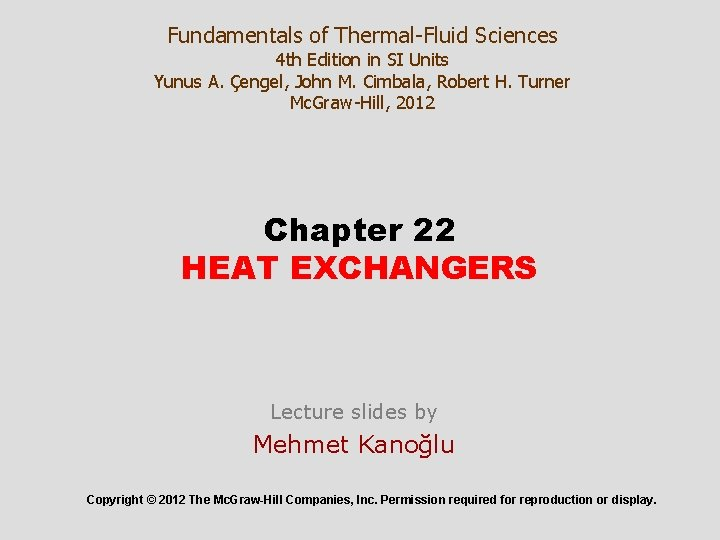Fundamentals of Thermal-Fluid Sciences 4 th Edition in SI Units Yunus A. Çengel, John M. Cimbala, Robert H. Turner Mc. Graw-Hill, 2012 Chapter 22 HEAT EXCHANGERS Lecture slides by Mehmet Kanoğlu Copyright © 2012 The Mc. Graw-Hill Companies, Inc. Permission required for reproduction or display.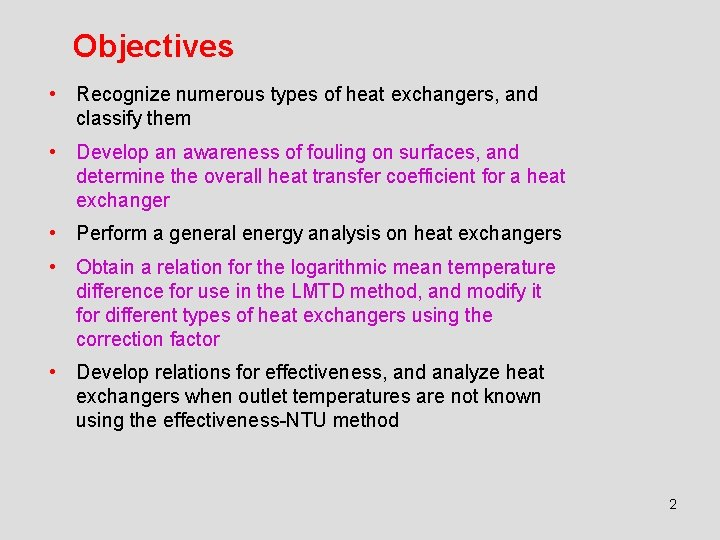Objectives • Recognize numerous types of heat exchangers, and classify them • Develop an awareness of fouling on surfaces, and determine the overall heat transfer coefficient for a heat exchanger • Perform a general energy analysis on heat exchangers • Obtain a relation for the logarithmic mean temperature difference for use in the LMTD method, and modify it for different types of heat exchangers using the correction factor • Develop relations for effectiveness, and analyze heat exchangers when outlet temperatures are not known using the effectiveness-NTU method 2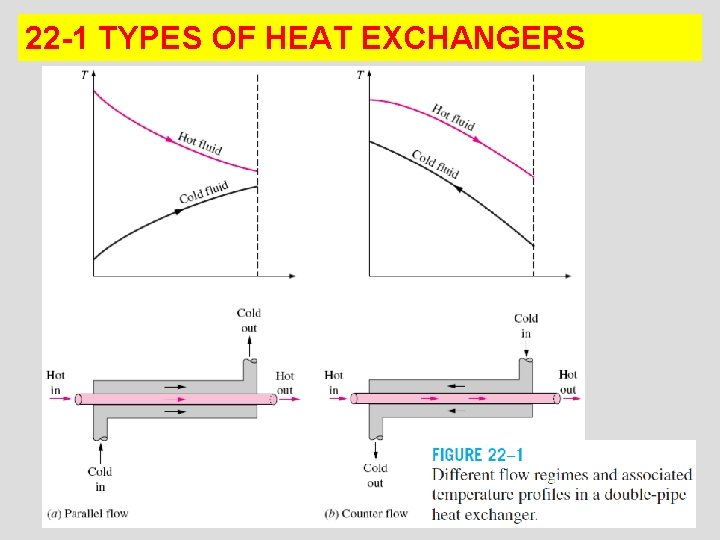22 -1 TYPES OF HEAT EXCHANGERS 3Compact heat exchanger: It has a large heat transfer surface area per unit volume (e. g. , car radiator, human lung). A heat exchanger with the area density > 700 m 2/m 3 is classified as being compact. Cross-flow: In compact heat exchangers, the two fluids usually move perpendicular to each other. The crossflow is further classified as unmixed and mixed flow. 4Shell-and-tube heat exchanger: The most common type of heat exchanger in industrial applications. They contain a large number of tubes (sometimes several hundred) packed in a shell with their axes parallel to that of the shell. Heat transfer takes place as one fluid flows inside the tubes while the other fluid flows outside the tubes through the shell. Shell-and-tube heat exchangers are further classified according to the number of shell and tube passes involved. 5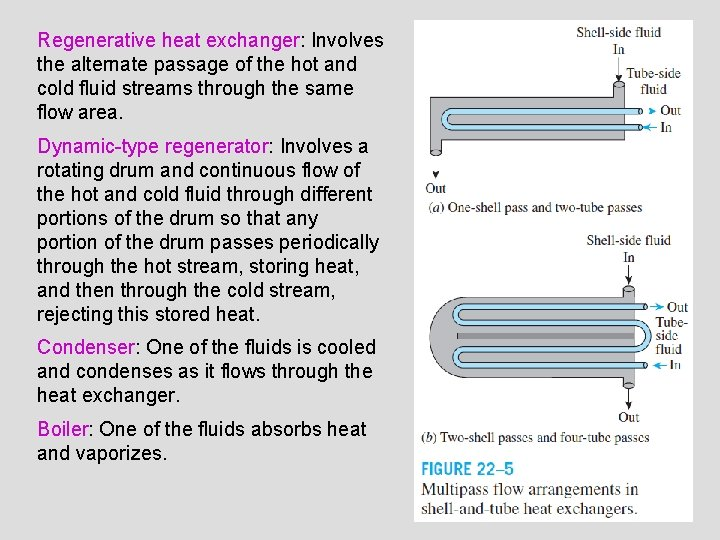Regenerative heat exchanger: Involves the alternate passage of the hot and cold fluid streams through the same flow area. Dynamic-type regenerator: Involves a rotating drum and continuous flow of the hot and cold fluid through different portions of the drum so that any portion of the drum passes periodically through the hot stream, storing heat, and then through the cold stream, rejecting this stored heat. Condenser: One of the fluids is cooled and condenses as it flows through the heat exchanger. Boiler: One of the fluids absorbs heat and vaporizes. 6Plate and frame (or just plate) heat exchanger: Consists of a series of plates with corrugated flat flow passages. The hot and cold fluids flow in alternate passages, and thus each cold fluid stream is surrounded by two hot fluid streams, resulting in very effective heat transfer. Well suited for liquid-to-liquid applications. A plate-and-frame liquid-to-liquid heat exchanger. 722 -2 THE OVERALL HEAT TRANSFER COEFFICIENT • A heat exchanger typically involves two flowing fluids separated by a solid wall. • Heat is first transferred from the hot fluid to the wall by convection, through the wall by conduction, and from the wall to the cold fluid again by convection. • Any radiation effects are usually included in the convection heat transfer coefficients. Thermal resistance network associated with heat transfer in a double-pipe heat exchanger. 8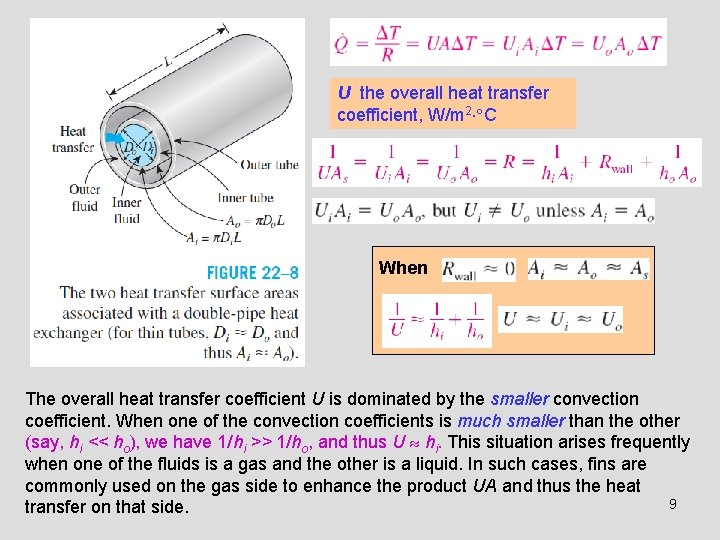U the overall heat transfer coefficient, W/m 2 C When The overall heat transfer coefficient U is dominated by the smaller convection coefficient. When one of the convection coefficients is much smaller than the other (say, hi << ho), we have 1/hi >> 1/ho, and thus U hi. This situation arises frequently when one of the fluids is a gas and the other is a liquid. In such cases, fins are commonly used on the gas side to enhance the product UA and thus the heat 9 transfer on that side.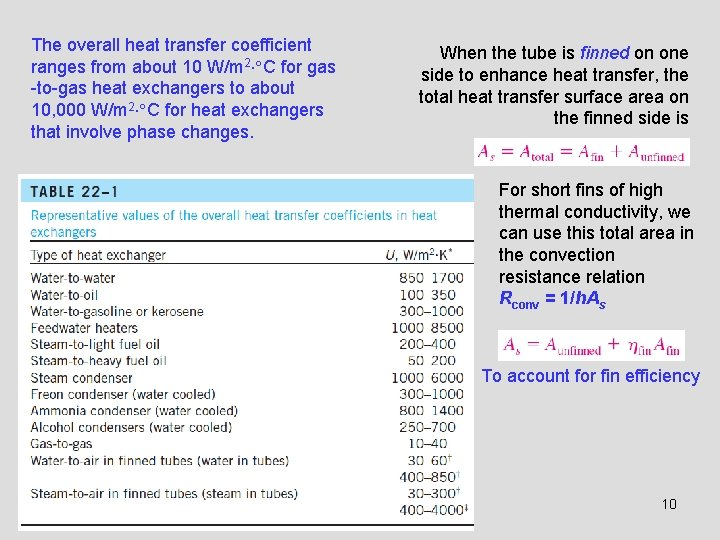The overall heat transfer coefficient ranges from about 10 W/m 2 C for gas -to-gas heat exchangers to about 10, 000 W/m 2 C for heat exchangers that involve phase changes. When the tube is finned on one side to enhance heat transfer, the total heat transfer surface area on the finned side is For short fins of high thermal conductivity, we can use this total area in the convection resistance relation Rconv = 1/h. As To account for fin efficiency 10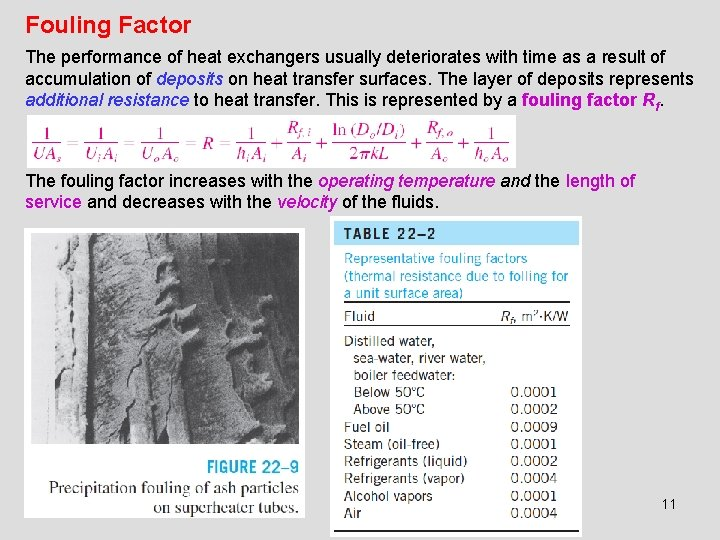Fouling Factor The performance of heat exchangers usually deteriorates with time as a result of accumulation of deposits on heat transfer surfaces. The layer of deposits represents additional resistance to heat transfer. This is represented by a fouling factor Rf. The fouling factor increases with the operating temperature and the length of service and decreases with the velocity of the fluids. 11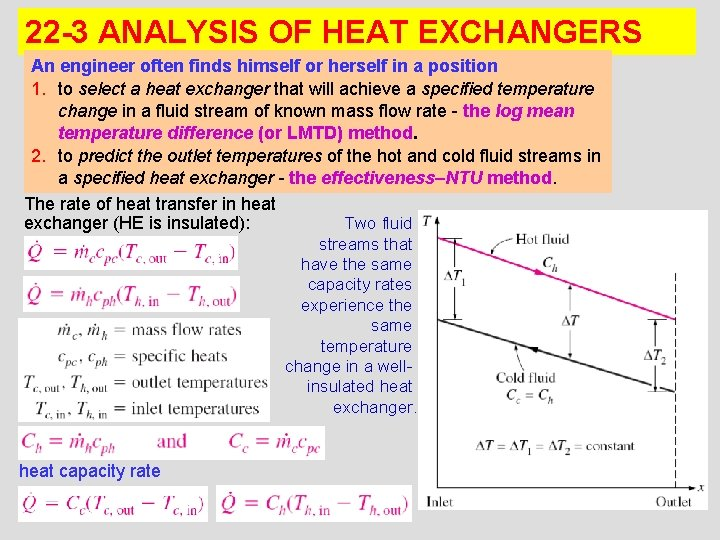22 -3 ANALYSIS OF HEAT EXCHANGERS An engineer often finds himself or herself in a position 1. to select a heat exchanger that will achieve a specified temperature change in a fluid stream of known mass flow rate - the log mean temperature difference (or LMTD) method. 2. to predict the outlet temperatures of the hot and cold fluid streams in a specified heat exchanger - the effectiveness–NTU method. The rate of heat transfer in heat exchanger (HE is insulated): Two fluid streams that have the same capacity rates experience the same temperature change in a wellinsulated heat exchanger. heat capacity rate 12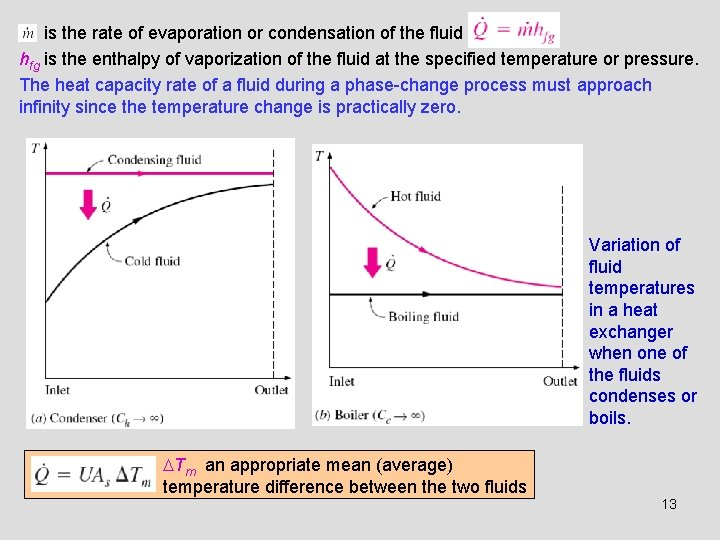is the rate of evaporation or condensation of the fluid hfg is the enthalpy of vaporization of the fluid at the specified temperature or pressure. The heat capacity rate of a fluid during a phase-change process must approach infinity since the temperature change is practically zero. Variation of fluid temperatures in a heat exchanger when one of the fluids condenses or boils. Tm an appropriate mean (average) temperature difference between the two fluids 13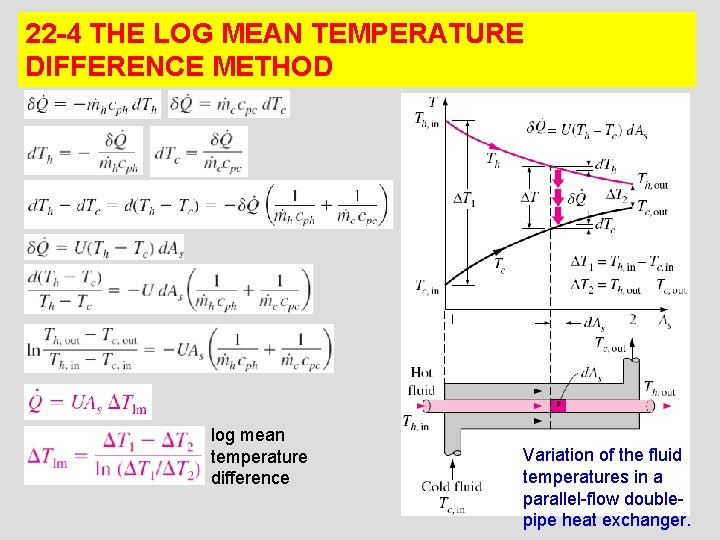22 -4 THE LOG MEAN TEMPERATURE DIFFERENCE METHOD log mean temperature difference Variation of the fluid temperatures in a parallel-flow double 14 pipe heat exchanger.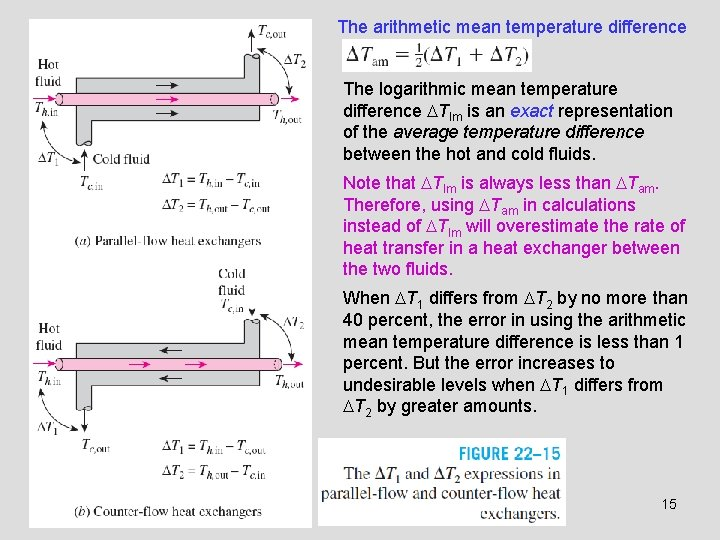The arithmetic mean temperature difference The logarithmic mean temperature difference Tlm is an exact representation of the average temperature difference between the hot and cold fluids. Note that Tlm is always less than Tam. Therefore, using Tam in calculations instead of Tlm will overestimate the rate of heat transfer in a heat exchanger between the two fluids. When T 1 differs from T 2 by no more than 40 percent, the error in using the arithmetic mean temperature difference is less than 1 percent. But the error increases to undesirable levels when T 1 differs from T 2 by greater amounts. 15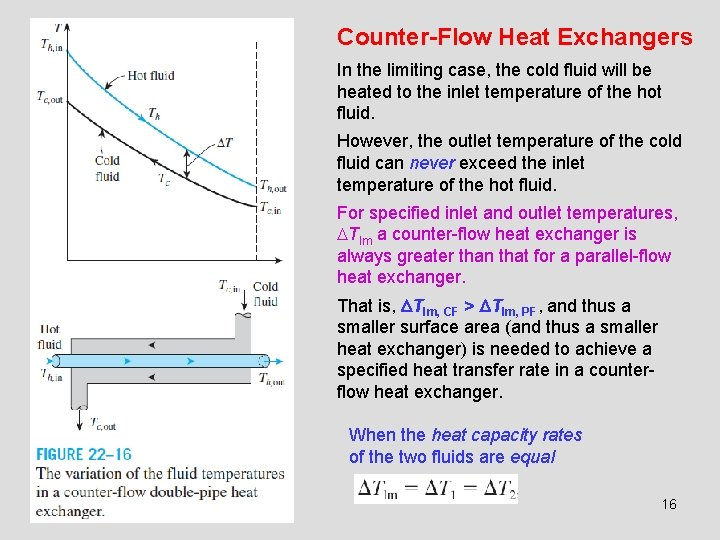Counter-Flow Heat Exchangers In the limiting case, the cold fluid will be heated to the inlet temperature of the hot fluid. However, the outlet temperature of the cold fluid can never exceed the inlet temperature of the hot fluid. For specified inlet and outlet temperatures, Tlm a counter-flow heat exchanger is always greater than that for a parallel-flow heat exchanger. That is, Tlm, CF > Tlm, PF, and thus a smaller surface area (and thus a smaller heat exchanger) is needed to achieve a specified heat transfer rate in a counterflow heat exchanger. When the heat capacity rates of the two fluids are equal 16Multipass and Cross-Flow Heat Exchangers: Use of a Correction Factor F correction factor depends on the geometry of the heat exchanger and the inlet and outlet temperatures of the hot and cold fluid streams. F for common cross-flow and shell-andtube heat exchanger configurations is given in the figure versus two temperature ratios P and R defined as 1 and 2 inlet and outlet T and t shell- and tube-side temperatures F = 1 for a condenser or boiler 17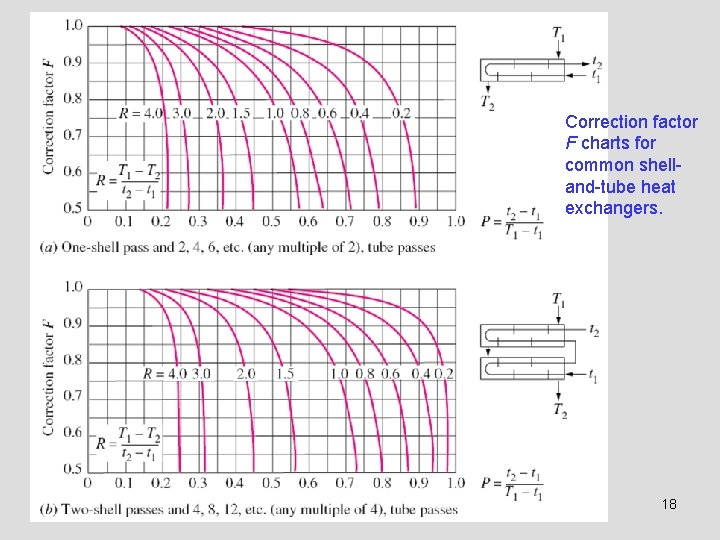Correction factor F charts for common shelland-tube heat exchangers. 18Correction factor F charts for common cross-flow heat exchangers. 19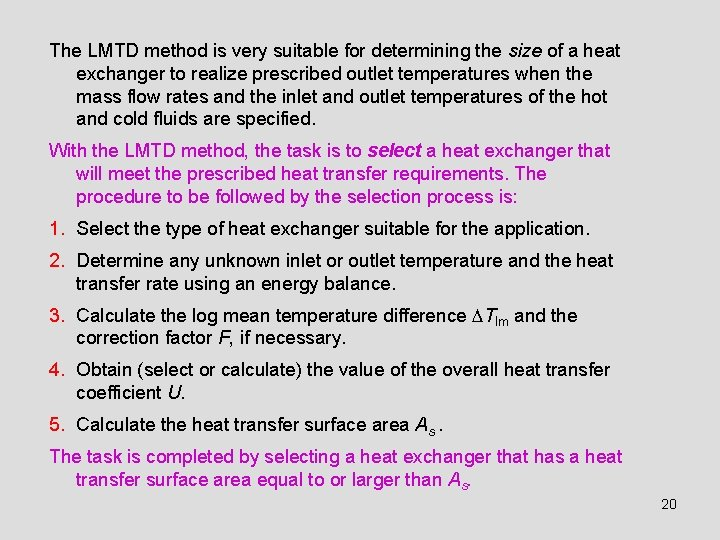The LMTD method is very suitable for determining the size of a heat exchanger to realize prescribed outlet temperatures when the mass flow rates and the inlet and outlet temperatures of the hot and cold fluids are specified. With the LMTD method, the task is to select a heat exchanger that will meet the prescribed heat transfer requirements. The procedure to be followed by the selection process is: 1. Select the type of heat exchanger suitable for the application. 2. Determine any unknown inlet or outlet temperature and the heat transfer rate using an energy balance. 3. Calculate the log mean temperature difference Tlm and the correction factor F, if necessary. 4. Obtain (select or calculate) the value of the overall heat transfer coefficient U. 5. Calculate the heat transfer surface area As. The task is completed by selecting a heat exchanger that has a heat transfer surface area equal to or larger than As. 20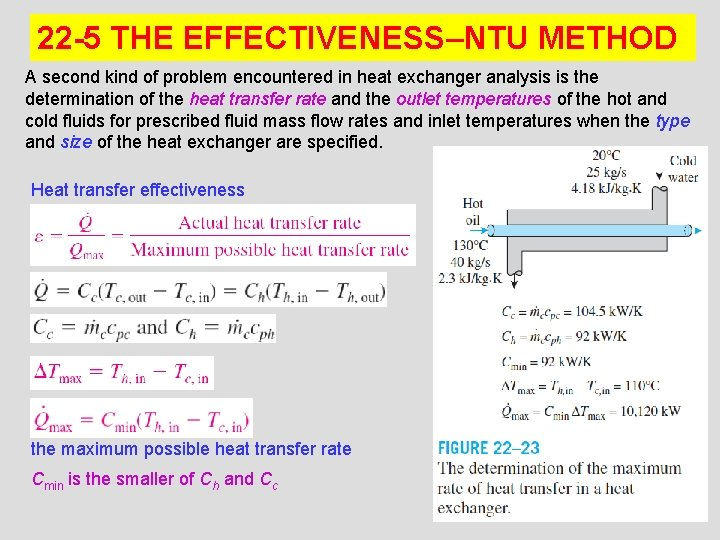22 -5 THE EFFECTIVENESS–NTU METHOD A second kind of problem encountered in heat exchanger analysis is the determination of the heat transfer rate and the outlet temperatures of the hot and cold fluids for prescribed fluid mass flow rates and inlet temperatures when the type and size of the heat exchanger are specified. Heat transfer effectiveness the maximum possible heat transfer rate Cmin is the smaller of Ch and Cc 21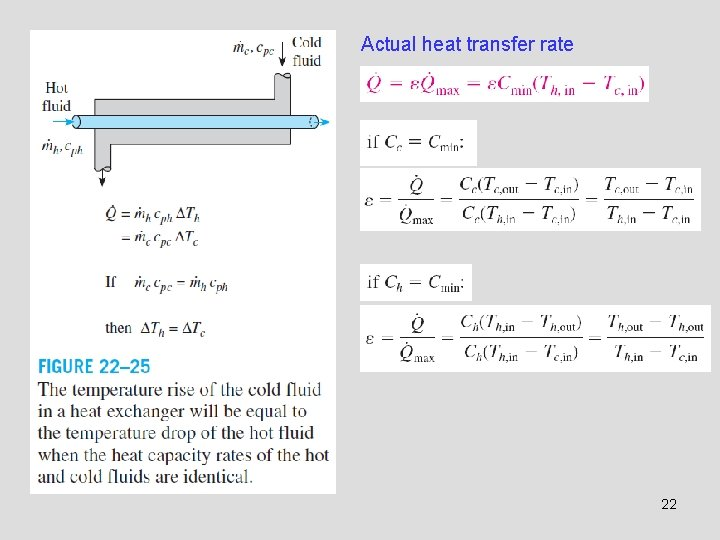Actual heat transfer rate 22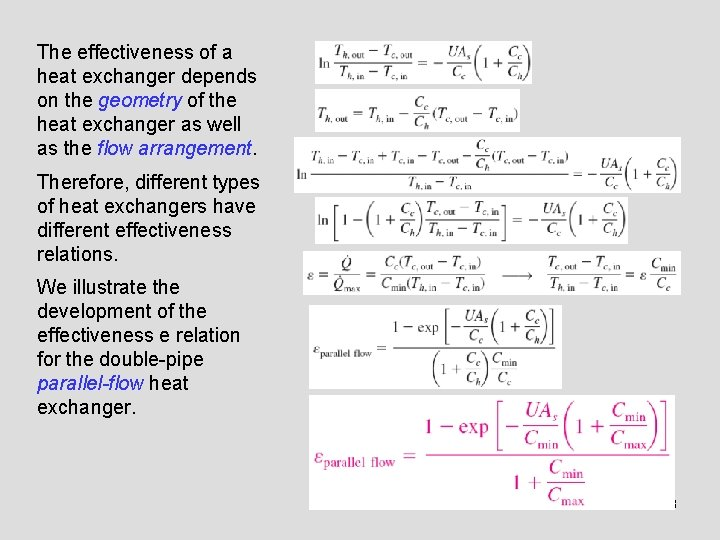The effectiveness of a heat exchanger depends on the geometry of the heat exchanger as well as the flow arrangement. Therefore, different types of heat exchangers have different effectiveness relations. We illustrate the development of the effectiveness e relation for the double-pipe parallel-flow heat exchanger. 23Effectiveness relations of the heat exchangers typically involve the dimensionless group UAs /Cmin. This quantity is called the number of transfer units NTU. capacity ratio For specified values of U and Cmin, the value of NTU is a measure of the surface area As. Thus, the larger the NTU, the larger the heat exchanger. The effectiveness of a heat exchanger is a function of the number of transfer units NTU and the capacity ratio c. 24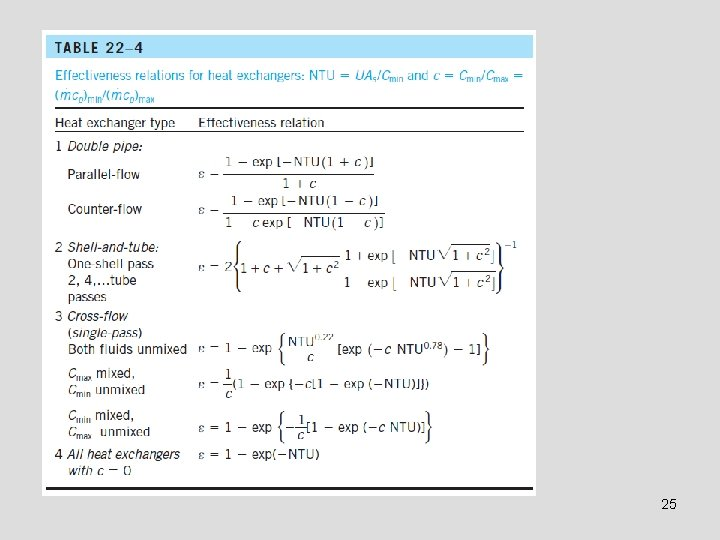25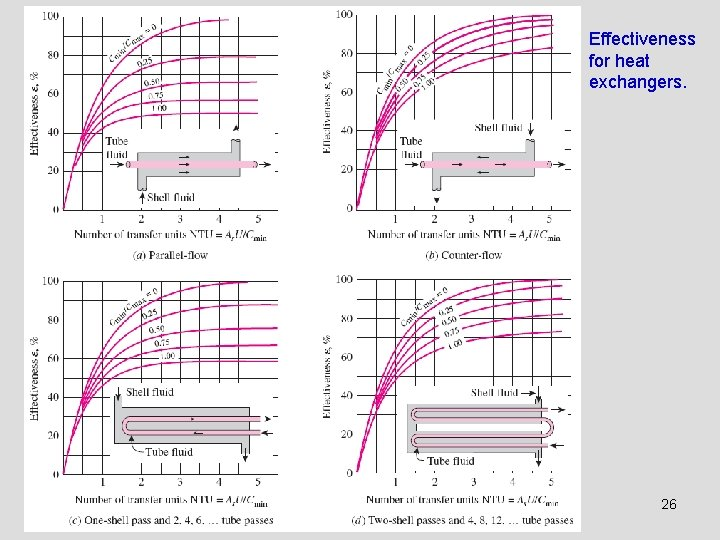Effectiveness for heat exchangers. 26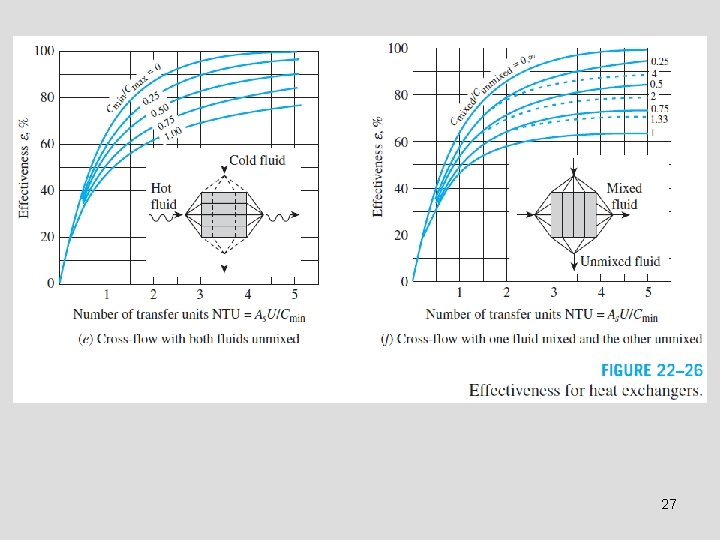27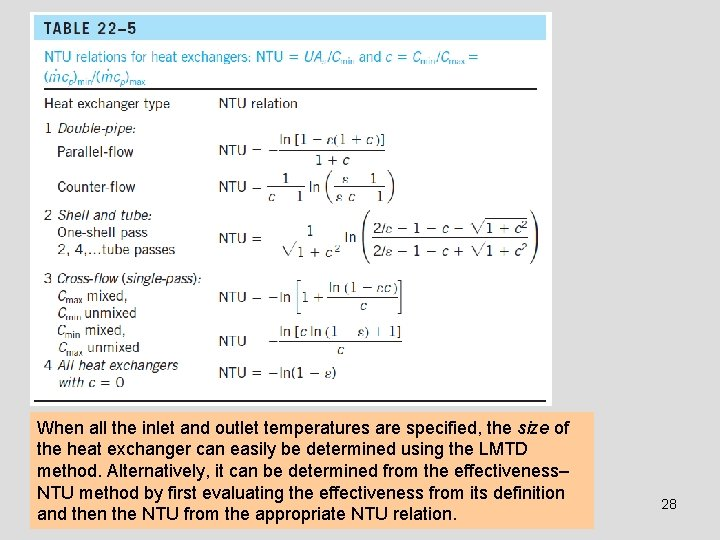When all the inlet and outlet temperatures are specified, the size of the heat exchanger can easily be determined using the LMTD method. Alternatively, it can be determined from the effectiveness– NTU method by first evaluating the effectiveness from its definition and then the NTU from the appropriate NTU relation. 28(e. g. , boiler, condenser) 29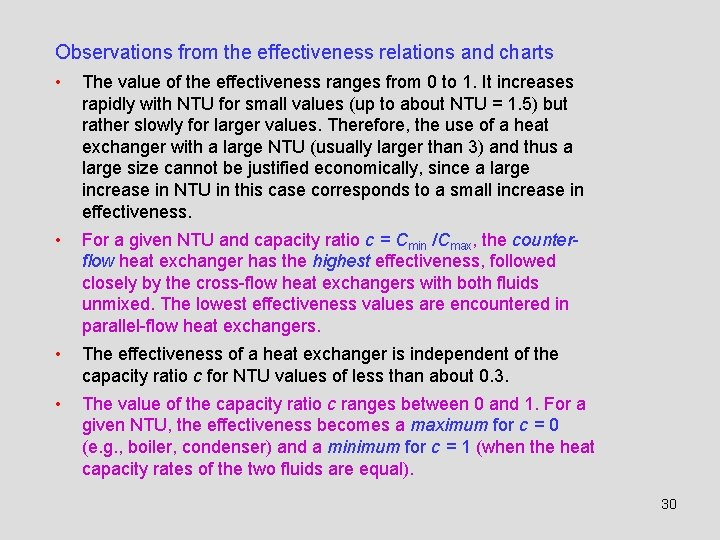Observations from the effectiveness relations and charts • The value of the effectiveness ranges from 0 to 1. It increases rapidly with NTU for small values (up to about NTU = 1. 5) but rather slowly for larger values. Therefore, the use of a heat exchanger with a large NTU (usually larger than 3) and thus a large size cannot be justified economically, since a large increase in NTU in this case corresponds to a small increase in effectiveness. • For a given NTU and capacity ratio c = Cmin /Cmax, the counterflow heat exchanger has the highest effectiveness, followed closely by the cross-flow heat exchangers with both fluids unmixed. The lowest effectiveness values are encountered in parallel-flow heat exchangers. • The effectiveness of a heat exchanger is independent of the capacity ratio c for NTU values of less than about 0. 3. • The value of the capacity ratio c ranges between 0 and 1. For a given NTU, the effectiveness becomes a maximum for c = 0 (e. g. , boiler, condenser) and a minimum for c = 1 (when the heat capacity rates of the two fluids are equal). 30Summary • Types of Heat Exchangers • The Overall Heat Transfer Coefficient ü Fouling factor • Analysis of Heat Exchangers • The Log Mean Temperature Difference Method ü Counter-Flow Heat Exchangers ü Multipass and Cross-Flow Heat Exchangers: Use of a Correction Factor • The Effectiveness–NTU Method 31